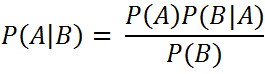`  I request GUEST WRITERS for this web site. Anyone interested please contact me at saravu2k2013@gmail.com Thanks  `
Showing posts with label Dependency Preservation. Show all posts
Showing posts with label Dependency Preservation. Show all posts

## Dependency preserving decomposition

Consider a relation R (A, B, C) with the following set of functional dependencies;
F = {A B, B C}.
Is the decomposition of R (A, B, C) into R1 (A, C) and R2 (B, C) a dependency preserving decomposition?

Solution:

The above said decomposition of R into R1 and R2 is a dependency preserving decomposition if (F1 U F2)+ = F+, where F1 is set of FDs hold by R1, F2 is set of FDs hold by R2, and F is the set of FDs hold by R. (F1 U F2)+ is the closure of (F1 U F2), and F+ is the closure of F.
Step 1: for R1, the non-trivial functional dependency is A C. Hence F1 = {A C}.
Step 2: for R2, the non-trivial functional dependency is B C. Hence, F2 = {B C}.
Step 3: find F1 U F2 and the closure of F1 U F2.

F1 U F2 = {A C, B C} ≠ F.
(F1 U F2)+ = {A C, B C}
F+ = {A B, B C, A C}
And, (F1 U F2)+ F.

The FD A B is not supported by (F1 U F2)+. Hence, the decomposition of R (A, B, C) into R1 (A, C) and R2 (B, C) is not a dependency preserving decomposition.

## Dependency preserving decomposition - Dependency preserving decomposition solved exercises - How to verify that a decomposition is dependency preserving? - Steps to find dependency preserving decomposition - Dependency preserving decomposition examples

Dependency preserving decomposition

Consider a relation R (A, B, C, D) with the following set of functional dependencies;
F = {A B, B C, and C D}.
Is the decomposition of R (A, B, C, D) into R1 (A, C, D) and R2 (B, C) a dependency preserving decomposition?

Solution:

The above said decomposition of R into R1 and R2 is a dependency preserving decomposition if (F1 U F2)+ = F+, where F1 is set of FDs hold by R1, F2 is set of FDs hold by R2, and F is the set of FDs hold by R. (F1 U F2)+ is the closure of (F1 U F2), and F+ is the closure of F.

Step 1: for R1, the derivable non-trivial functional dependency is, C D. Hence, F1 = {C D}
Step 2: for R2, the derivable non-trivial functional dependency is, B C. Hence, F2 = {B C}
(F1 U F2) = ({C D} U {B C}) = {C D, B C} ≠ F.
(F1 U F2)+ = {C D, B C, B D}
F+ = {A B, B C, C D, A C, A D, B D}
Hence, (F1 U F2)+ ≠ F+

The FD A B is not supported by (F1 U F2)+. Hence, the decomposition of R (A, B, C, D) into R1 (A, C, D) and R2 (B, C) is not a dependency preserving decomposition.

## Dependency preserving decomposition - Dependency preserving decomposition solved exercises - How to verify that a decomposition is dependency preserving? - Steps to find dependency preserving decomposition - Dependency preserving decomposition examples

Dependency preserving decomposition
Consider a relation R (A, B, C, D) with the following set of functional dependencies;
F = {A B, B C, and C D}.
Is the decomposition of R (A, B, C, D) into R1 (A, B, C) and R2 (C, D) a dependency preserving decomposition?

Solution:

The above said decomposition of R into R1 and R2 is a dependency preserving decomposition if (F1 U F2)+ = F+, where F1 is set of FDs hold by R1, F2 is set of FDs hold by R2, and F is the set of FDs hold by R.

Step 1: for R1, the derivable non-trivial functional dependencies are, A B, and B C. Hence, F1 = {A B, B C}

Step 2: for R2, the derivable non-trivial functional dependency is, C D. Hence, F2 = {C D}

(F1 U F2) = ({A B, B C} U {C D}) = {A B, B C, C D} = F.

F1 U F2 and F both have same set of functional dependencies. Hence, the decomposition of R (A, B, C, D) into R1 (A, B, C) and R2 (C, D) a dependency preserving decomposition.

Go back to Dependency preserving decomposition - solved exercises/examples page.

## List of solved exercises/examples for Dependency preserving decomposition:

• Dependency preserving decomposition – Solved Exercise/Example 4

• Dependency preserving decomposition – Solved Exercise/Example 5

• Dependency preserving decomposition – Solved Exercise/Example 6

• Dependency preserving decomposition – Solved Exercise/Example 7

• Dependency preserving decomposition – Solved Exercise/Example 8

• Dependency preserving decomposition – Solved Exercise/Example 9

• Dependency preserving decomposition – Solved Exercise/Example 10

### Simple introduction to Naive Bayes classifier

Simple introduction to Naive Bayes classifier What is Naive Bayes Classifier? A Naive Bayes classifier is a probabilistic classifier ...